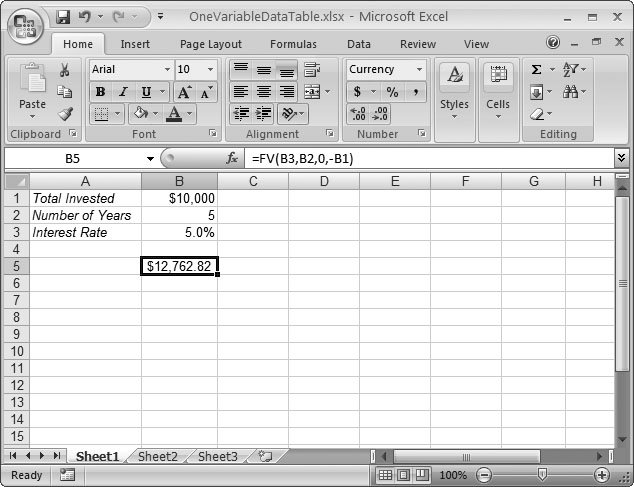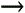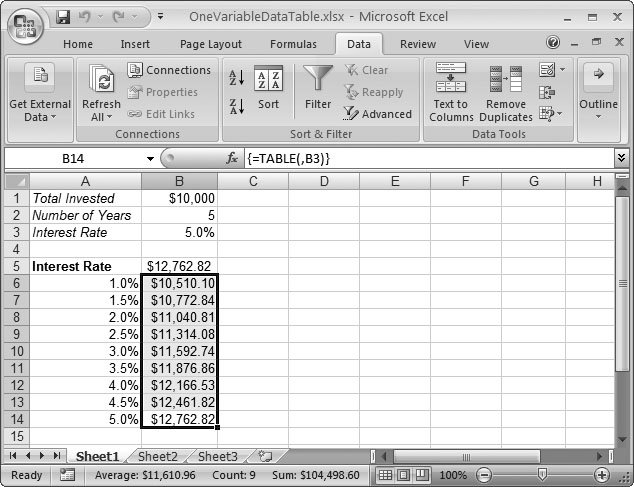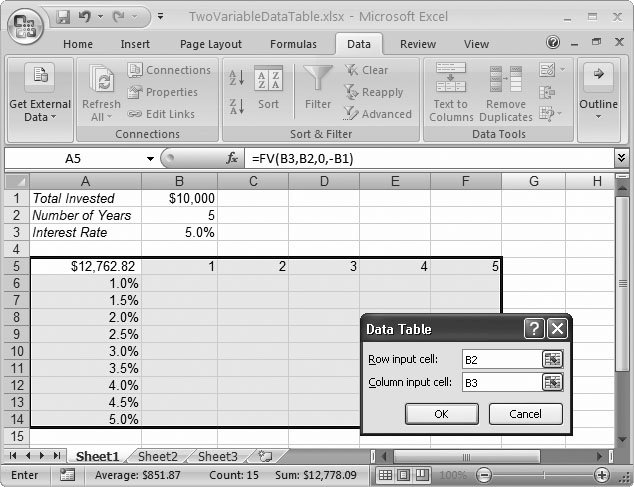# Section 13.3. Variable Data Tables

### 13.3. Variable Data Tables

When you create a formula, you generate a single result based on the operators, functions, and data that you used. This situation makes sense when you're nailing down last year's profit-loss report, but it's not always as handy when you're making projections for the future . In these cases, it's often helpful to compare several possibilities. One tool you can use is a variable data table .

A variable data table is simply a table that shows multiple results, based on different source data. You could use a variable data table to see how the return of an investment varies based on different interest rates. Because Excel shows all the results side by side, you can quickly compare them.

You could create your own comparison table by hand without too much trouble. In fact, using the power of relative references, you could create one formula and copy it into several cells to quickly create a table without needing any formula tweaking. Some Excel fans prefer this approach. However, when you use variable data tables you save more than a few keystrokes.

Note: Variable data tables have nothing in common with the similarly named table feature , which you'll learn about in Chapter 14.

#### 13.3.1. Creating a One-Variable Data Table

A one-variable data table provides a single column of results. It's called one-variable because there's only one input value that changes. For example, if you want to compare how an investment payoff changes based on interest rates, you can create a one-variable data table that uses the interest rate as the changing variable.

Here's how you create a one-variable data table:

1. Create a sample formula that performs the calculation you plan to use .

When you create this formula, make sure that you use a cell reference for the value you want to change, not a literal value. Otherwise, Excel can't change the value to calculate the different possibilities.

In the example in Figure 13-13, the formula uses the FV() financial function (Section 10.2) to calculate the future value of an investment. The variable is the rate argument, which is stored in cell B3.Figure 13-13. Before you can create a data table, you need to start with a formula that calculates the result, using cell references. In this example, the formula is in cell B5, and it calculates the value of a \$10,000 investment after 5 years at five percent interest.

2. Set up the table. To do so, create a column that has each value you want to test .

In this example, you need to create a column with the different interest rate possibilities. The calculated results appear in the column on the right. You may find getting the correct arrangement a little tricky. You need to make sure the formula you want to modify is just above the column where the results will appear, as shown in Figure 13-14.Figure 13-14. In the Data Table dialog box, specify the cell that has the changing value, and then click OK.

3. Generate the table by selecting the whole table and then choosing DataData ToolsWhat-If AnalysisData Table .

The Data Table dialog box appears (Figure 13-14).

4. In the "Column input cell" box, type the address of the cell that has the value you want to change .

This part is tricky. Excel needs to figure out which part of the formula it's supposed to modify to generate the different results that appear in the table. In this example, the formula's = FV(B3,B2,0,-B1) . The cell B3 supplies the rate (currently 5 percent). This rate is the piece of information that needs to change, so you should enter B3 in the "Column input cell" text box. (When Excel generates the data table it looks at the first column, gets the new rate value, and substitutes this value into the formula where the reference B3 was previously.)

5. Click OK to create the table .

Excel fills in all the different resultsin this example, the value of the investment based on different interest rates (Figure 13-15). If you actually look at the content of each cell, you'll find that it uses an array formula with the TABLE() function to work its magic.

#### 13.3.2. Creating a Two-Variable Data Table

It's almost as easy to create a two-variable data table, where two different values change. In this case, you place one set of changing values in the column on the left. You place the other set of changing values in the row on the top. Figure 13-16 shows an example.Figure 13-15. The end result of a one-variable data table: a table of investment profits based on different interest rates.Figure 13-16. This data table studies how an investment performs for different interest rates and different investment periods (in years). The row of values B5 to F5 has the number of years, while the column of values A6 to A14 has the interest rate. The formula needs to be in the top-left corner of the table.

Next, select the whole table and choose DataData ToolsWhat-If AnalysisData Table. This time, fill out both text boxes (the top row provides an investment period value in place of cell B2, and the leftmost cell provides the interest rate in place of cell B3. When you click OK, Excel generates the complete table (Figure 13-17).Figure 13-17. The final table shows how the money you make changes based on the time you wait and the interest rate.

Tip: For some more sophisticated ways to perform what-if analysis, you can read about scenarios and goal seeking in Chapter 20.Excel 2007[c] The Missing Manual
ISBN: 596527594
EAN: N/A
Year: 2007
Pages: 173